#0
0
01. 云栖社区>
2. 博客>
3. 正文

## 如何用Python处理自然语言？（Spacy与Word Embedding）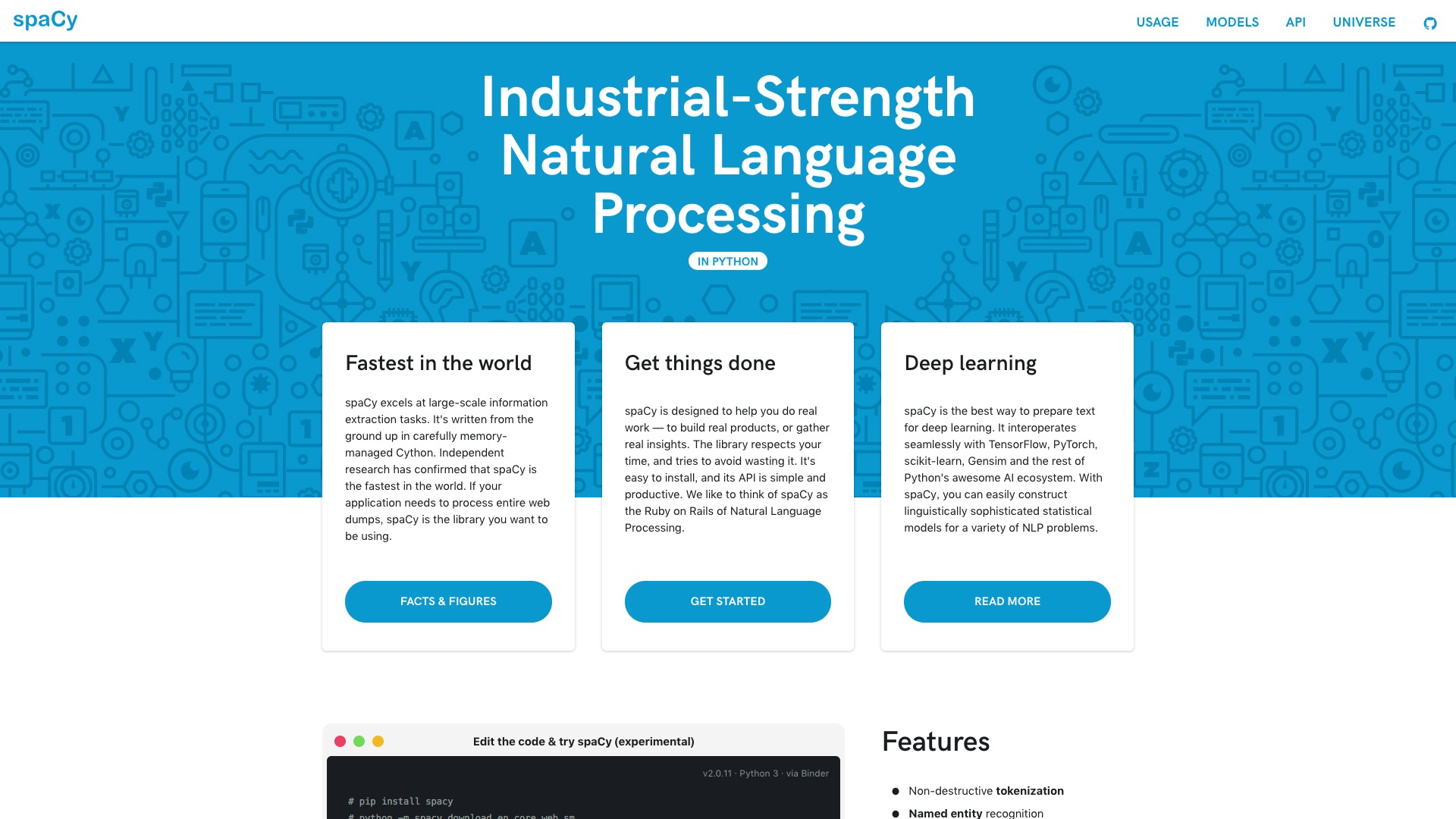# 盲维

To the one with a hammer, everything looks like a nail. （手中有锤，看什么都像钉）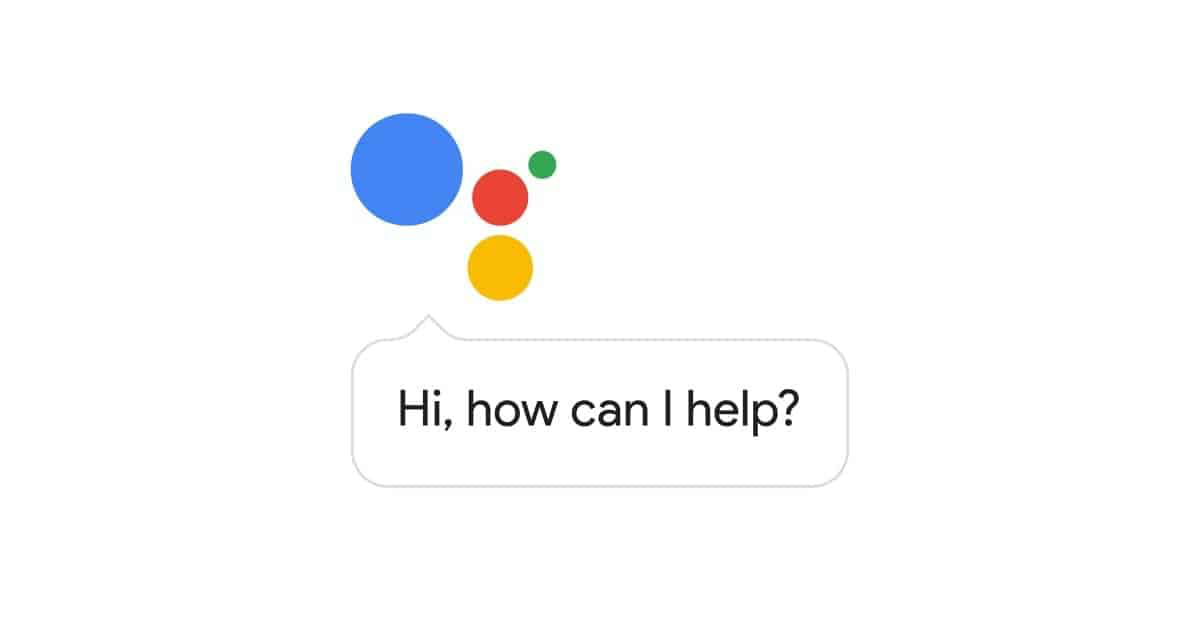# 工具

Spacy 的 Slogan，是这样的：

Industrial-Strength Natural Language Processing. （工业级别的自然语言处理）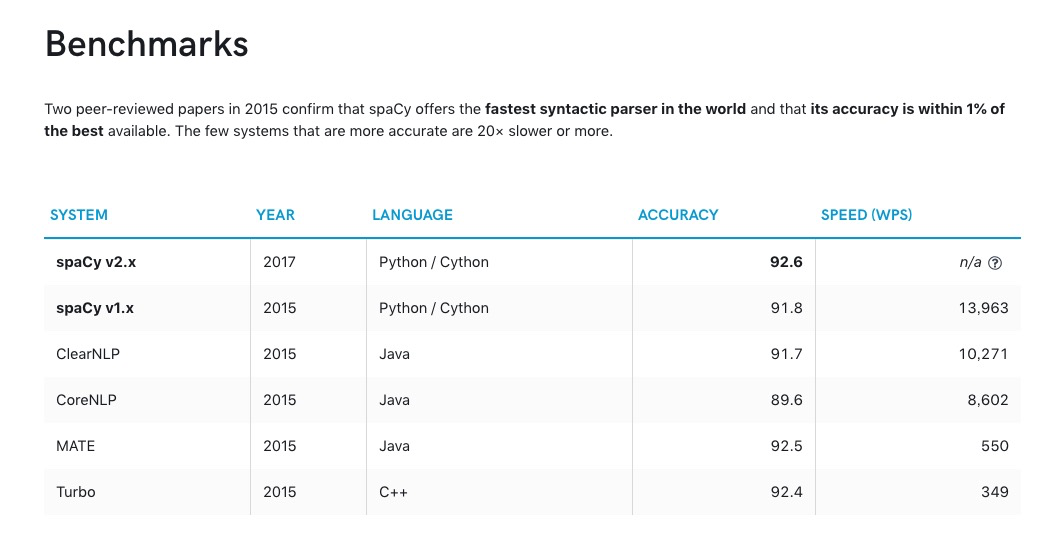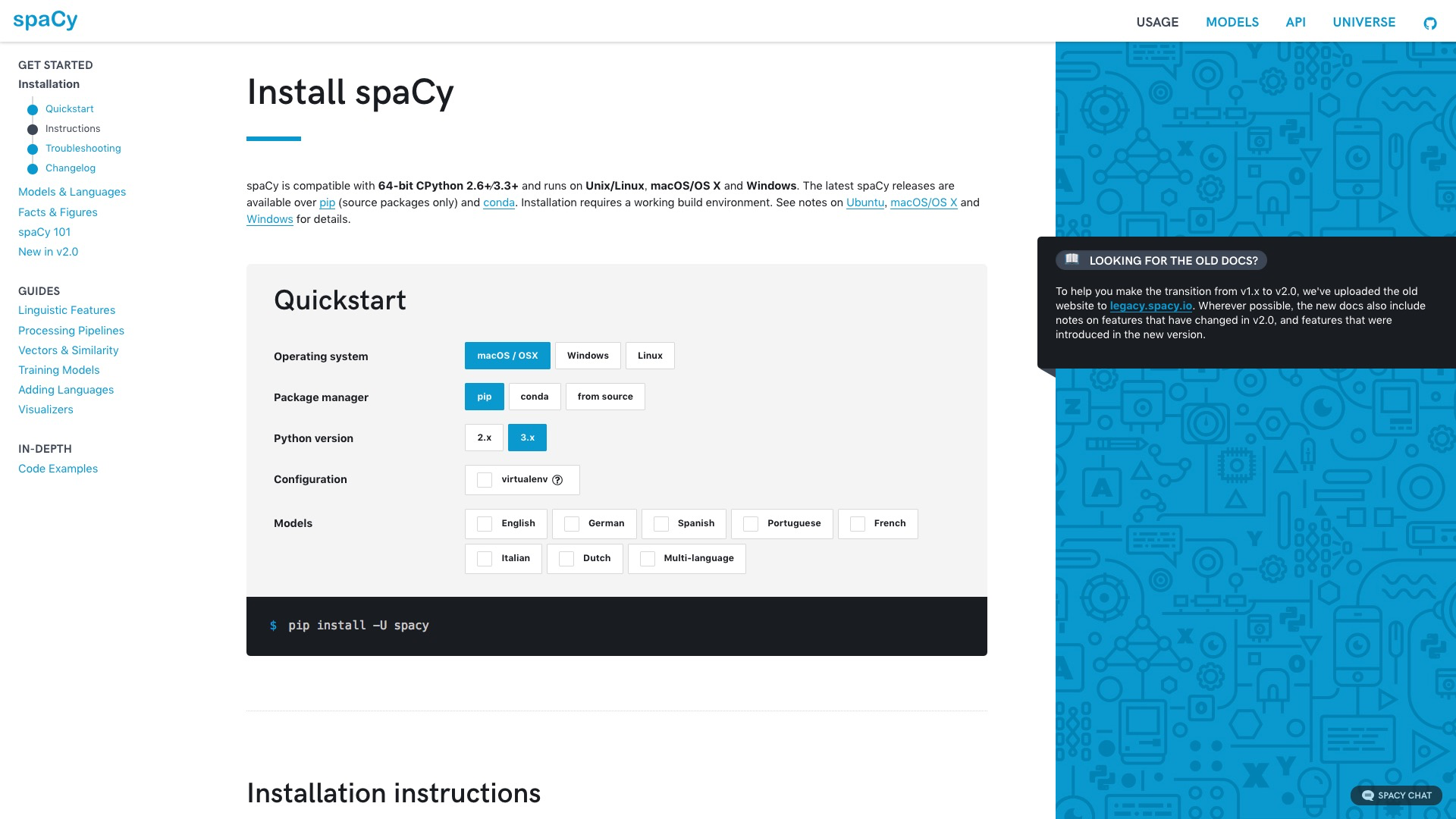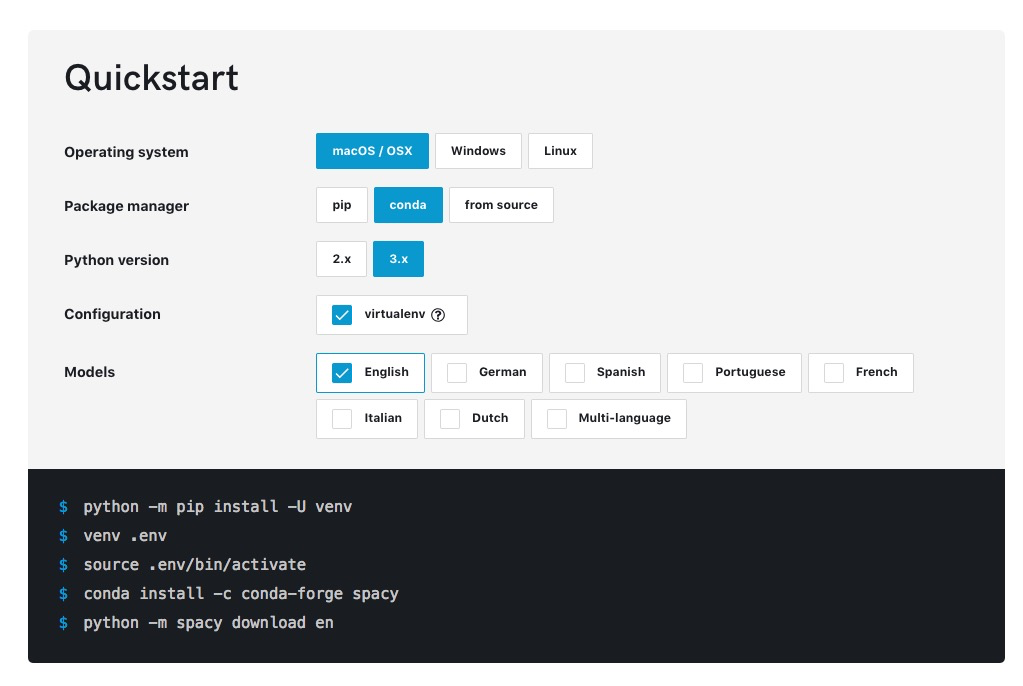Spacy的功能有很多。

• 词性分析
• 命名实体识别
• 依赖关系刻画
• 词嵌入向量的近似度计算
• 词语降维和可视化

# 环境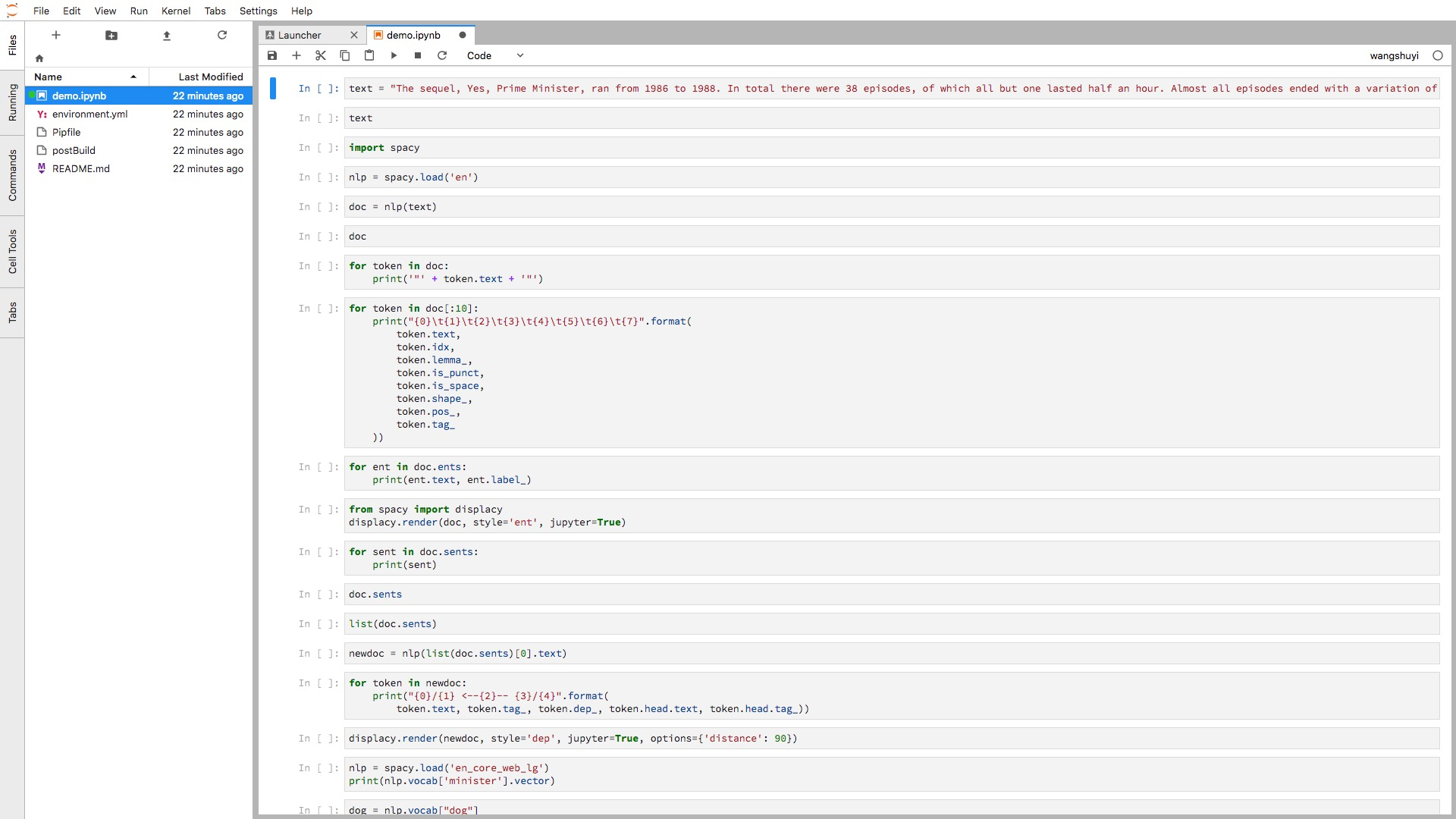• 代码单元直接鼠标拖动；
• 一个浏览器标签，可打开多个Notebook，而且分别使用不同的Kernel；
• 提供实时渲染的Markdown编辑器；
• 完整的文件浏览器；
• CSV数据文件快速浏览
• ……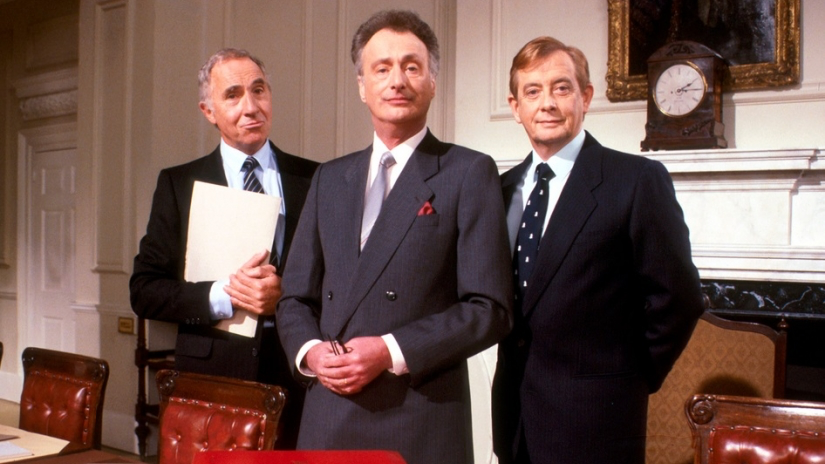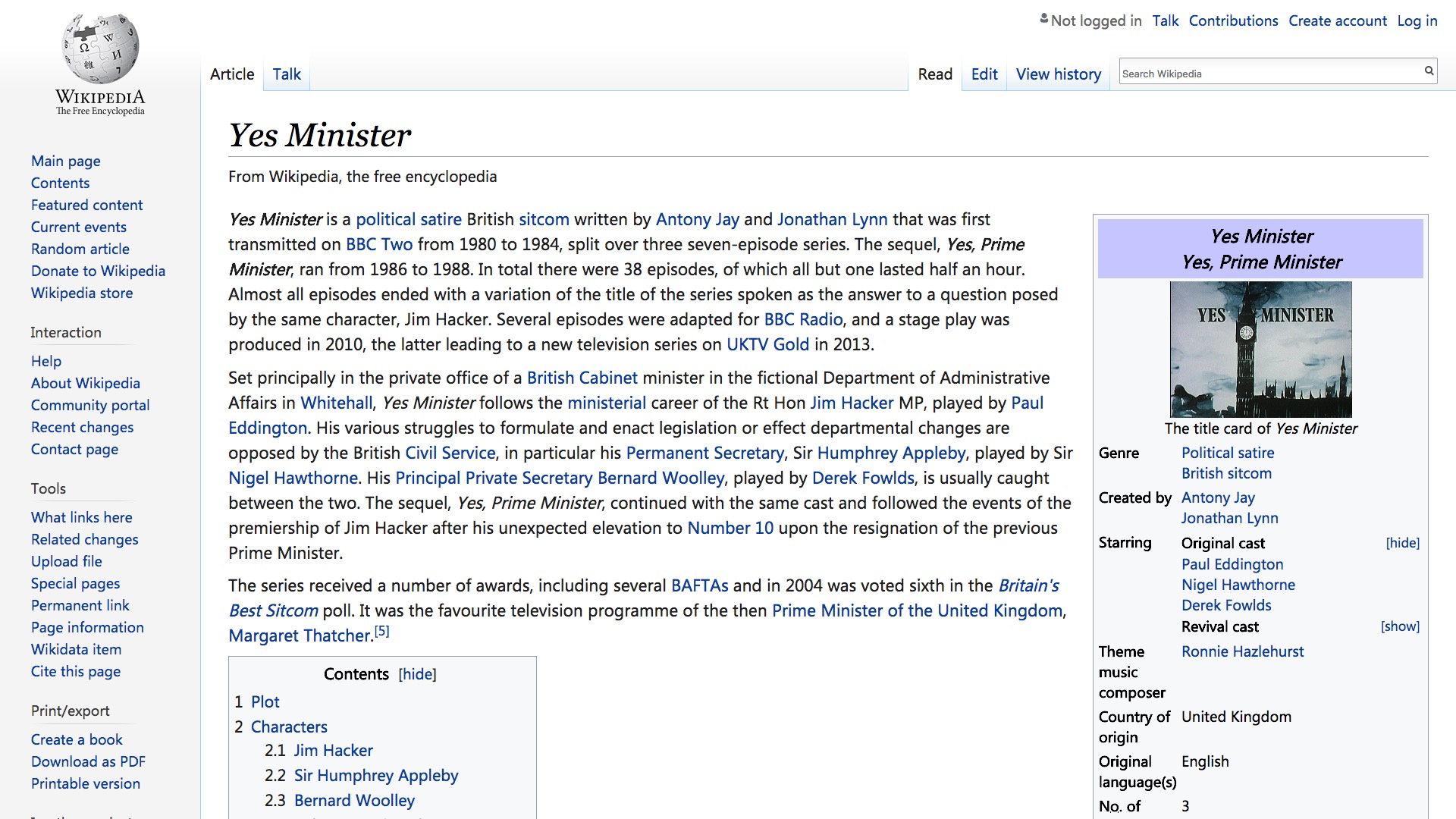# 实践

``````text = "The sequel, Yes, Prime Minister, ran from 1986 to 1988. In total there were 38 episodes, of which all but one lasted half an hour. Almost all episodes ended with a variation of the title of the series spoken as the answer to a question posed by the same character, Jim Hacker. Several episodes were adapted for BBC Radio, and a stage play was produced in 2010, the latter leading to a new television series on UKTV Gold in 2013."
``````

``````text
``````
``````'The sequel, Yes, Prime Minister, ran from 1986 to 1988. In total there were 38 episodes, of which all but one lasted half an hour. Almost all episodes ended with a variation of the title of the series spoken as the answer to a question posed by the same character, Jim Hacker. Several episodes were adapted for BBC Radio, and a stage play was produced in 2010, the latter leading to a new television series on UKTV Gold in 2013.'
``````

``````import spacy
``````

``````nlp = spacy.load('en')
``````

``````doc = nlp(text)
``````

``````doc
``````
``````The sequel, Yes, Prime Minister, ran from 1986 to 1988. In total there were 38 episodes, of which all but one lasted half an hour. Almost all episodes ended with a variation of the title of the series spoken as the answer to a question posed by the same character, Jim Hacker. Several episodes were adapted for BBC Radio, and a stage play was produced in 2010, the latter leading to a new television series on UKTV Gold in 2013.
``````

``````for token in doc:
print('"' + token.text + '"')
``````

``````"The"
"sequel"
","
"Yes"
","
"Prime"
"Minister"
","
"ran"
"from"
"1986"
"to"
"1988"
"."
"In"
"total"
"there"
"were"
"38"
"episodes"
","
"of"
"which"
"all"
"but"
"one"
"lasted"
"half"
"an"
"hour"
"."
"Almost"
"all"
"episodes"
"ended"
"with"
"a"
"variation"
"of"
"the"
"title"
"of"
"the"
"series"
"spoken"
"as"
"the"
"to"
"a"
"question"
"posed"
"by"
"the"
"same"
"character"
","
"Jim"
"Hacker"
"."
"Several"
"episodes"
"were"
"for"
"BBC"
","
"and"
"a"
"stage"
"play"
"was"
"produced"
"in"
"2010"
","
"the"
"latter"
"to"
"a"
"new"
"television"
"series"
"on"
"UKTV"
"Gold"
"in"
"2013"
"."
``````

• 文本
• 索引值（即在原文中的定位）
• 词元(lemma)
• 是否为标点符号
• 是否为空格
• 词性
• 标记
``````for token in doc[:10]:
print("{0}\t{1}\t{2}\t{3}\t{4}\t{5}\t{6}\t{7}".format(
token.text,
token.idx,
token.lemma_,
token.is_punct,
token.is_space,
token.shape_,
token.pos_,
token.tag_
))
``````

``````The 0   the False   False   Xxx DET DT
sequel  4   sequel  False   False   xxxx    NOUN    NN
,   10  ,   True    False   ,   PUNCT   ,
Yes 12  yes False   False   Xxx INTJ    UH
,   15  ,   True    False   ,   PUNCT   ,
Prime   17  prime   False   False   Xxxxx   PROPN   NNP
Minister    23  minister    False   False   Xxxxx   PROPN   NNP
,   31  ,   True    False   ,   PUNCT   ,
ran 33  run False   False   xxx VERB    VBD
from    37  from    False   False   xxxx    ADP IN
``````

``````for ent in doc.ents:
print(ent.text, ent.label_)
``````
``````1986 to 1988 DATE
38 CARDINAL
one CARDINAL
half an hour TIME
Jim Hacker PERSON
2010 DATE
UKTV Gold ORG
2013 DATE
``````

``````from spacy import displacy
displacy.render(doc, style='ent', jupyter=True)
``````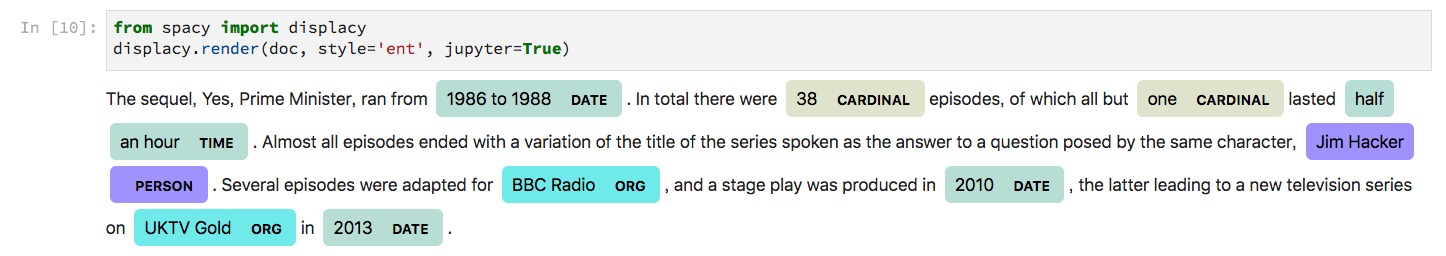``````for sent in doc.sents:
print(sent)
``````
``````The sequel, Yes, Prime Minister, ran from 1986 to 1988.
In total there were 38 episodes, of which all but one lasted half an hour.
Almost all episodes ended with a variation of the title of the series spoken as the answer to a question posed by the same character, Jim Hacker.
Several episodes were adapted for BBC Radio, and a stage play was produced in 2010, the latter leading to a new television series on UKTV Gold in 2013.
``````

``````doc.sents
``````
``````<generator at 0x116e95e18>
``````

``````list(doc.sents)
``````
``````[The sequel, Yes, Prime Minister, ran from 1986 to 1988.,
In total there were 38 episodes, of which all but one lasted half an hour.,
Almost all episodes ended with a variation of the title of the series spoken as the answer to a question posed by the same character, Jim Hacker.,
Several episodes were adapted for BBC Radio, and a stage play was produced in 2010, the latter leading to a new television series on UKTV Gold in 2013.]
``````

``````newdoc = nlp(list(doc.sents).text)
``````

``````for token in newdoc:
print("{0}/{1} <--{2}-- {3}/{4}".format(
``````
``````The/DT <--det-- sequel/NN
sequel/NN <--nsubj-- ran/VBD
,/, <--punct-- sequel/NN
Yes/UH <--intj-- sequel/NN
,/, <--punct-- sequel/NN
Prime/NNP <--compound-- Minister/NNP
Minister/NNP <--appos-- sequel/NN
,/, <--punct-- sequel/NN
ran/VBD <--ROOT-- ran/VBD
from/IN <--prep-- ran/VBD
1986/CD <--pobj-- from/IN
to/IN <--prep-- from/IN
1988/CD <--pobj-- to/IN
./. <--punct-- ran/VBD
``````

``````displacy.render(newdoc, style='dep', jupyter=True, options={'distance': 90})
``````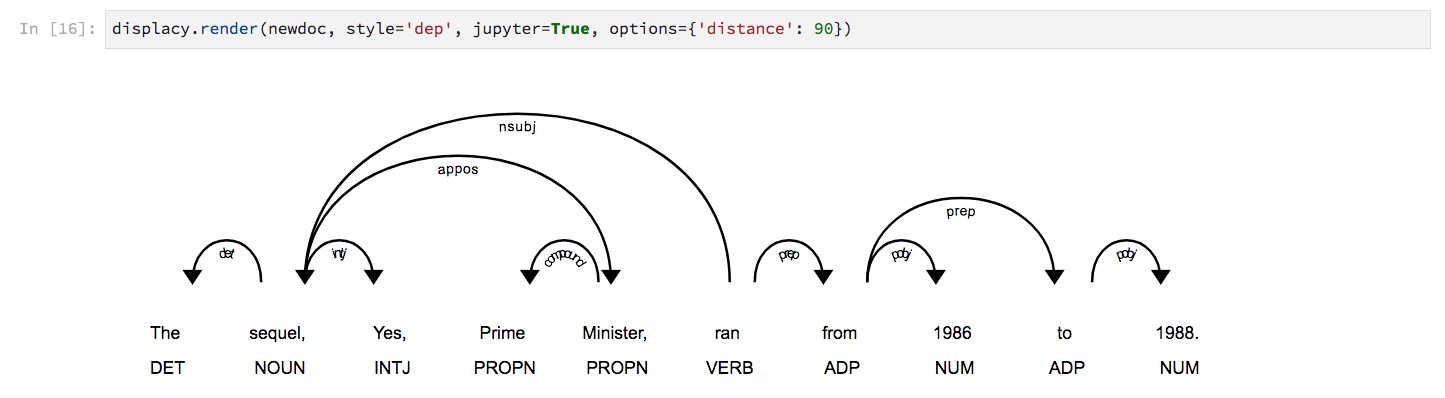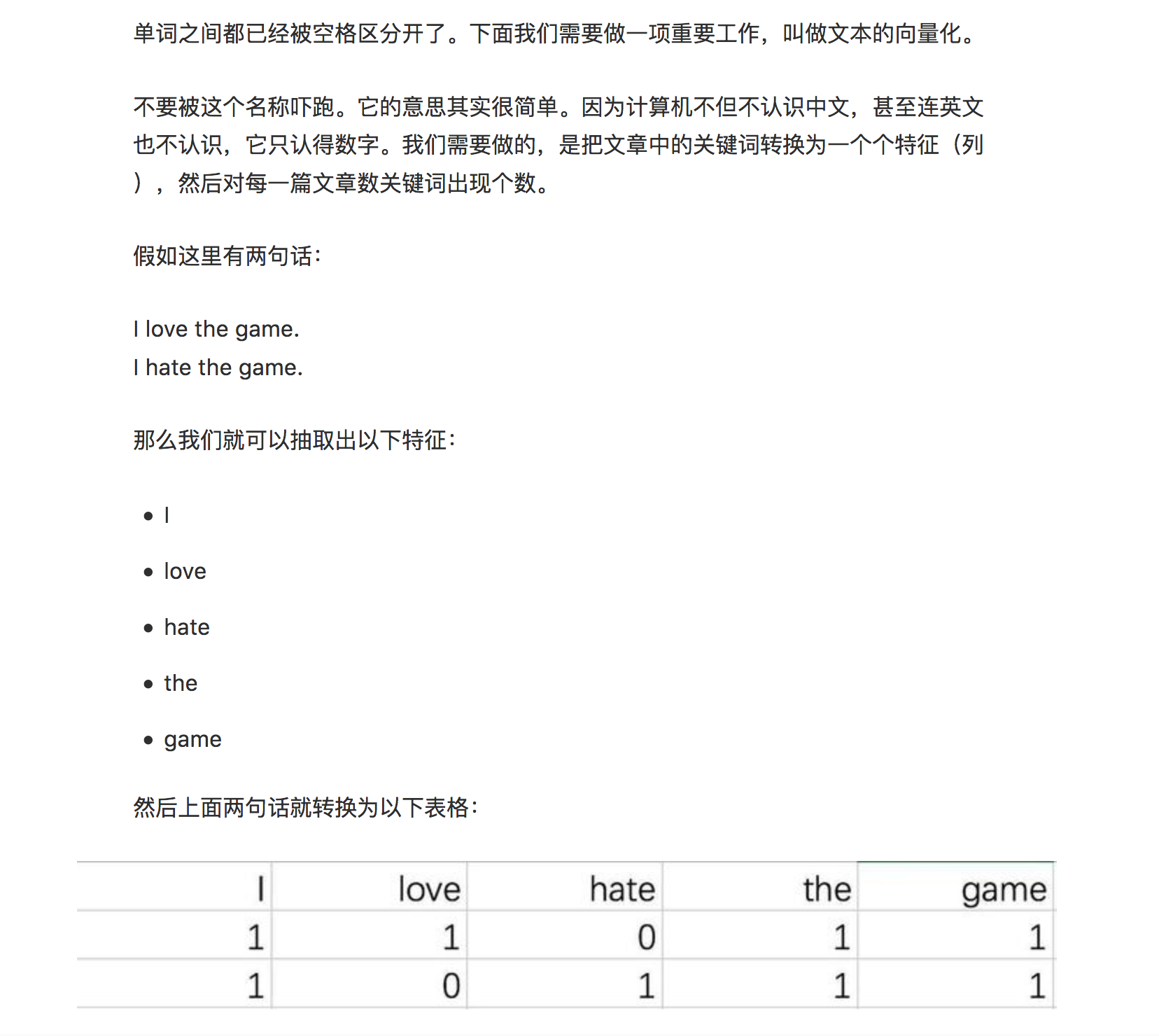``````? - woman = king - queen
``````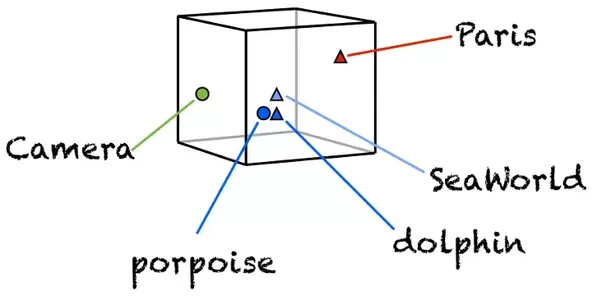``````nlp = spacy.load('en_core_web_lg')
``````

``````print(nlp.vocab['minister'].vector)
``````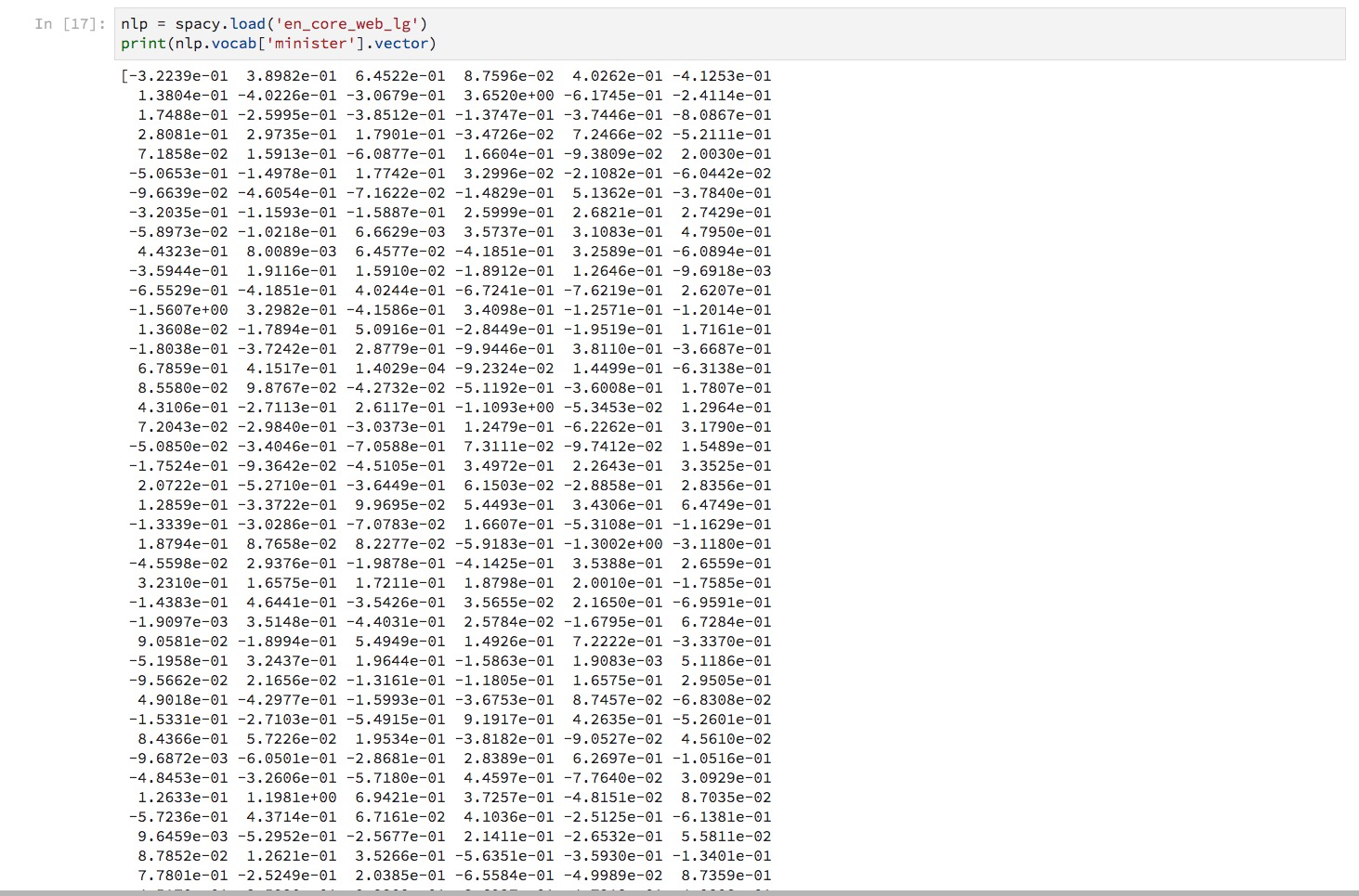``````dog = nlp.vocab["dog"]
cat = nlp.vocab["cat"]
apple = nlp.vocab["apple"]
orange = nlp.vocab["orange"]
``````

``````dog.similarity(cat)
``````
``````0.80168545
``````

``````dog.similarity(apple)
``````
``````0.26339027
``````

“狗”和“橘子”呢？

``````dog.similarity(orange)
``````
``````0.2742508
``````

``````apple.similarity(orange)
``````
``````0.5618917
``````

``````from scipy.spatial.distance import cosine
``````

``````1 - cosine(dog.vector, cat.vector)
``````
``````0.8016855120658875
``````

``````def vector_similarity(x, y):
return 1 - cosine(x, y)
``````

``````vector_similarity(dog.vector, apple.vector)
``````
``````0.2633902430534363
``````

``````? - woman = king - queen
``````

``````guess_word = king - queen + woman
``````

``````def make_guess_word(words):
[first, second, third] = words
return nlp.vocab[first].vector - nlp.vocab[second].vector + nlp.vocab[third].vector
``````

``````def get_similar_word(words, scope=nlp.vocab):

guess_word = make_guess_word(words)

similarities = []

for word in scope:
if not word.has_vector:
continue

similarity = vector_similarity(guess_word, word.vector)
similarities.append((word, similarity))

similarities = sorted(similarities, key=lambda item: -item)
print([word.text for word in similarities[:10]])
``````

``````? - woman = king - queen
``````

``````guess_word = king - queen + woman
``````

``````words = ["king", "queen", "woman"]
``````

``````get_similar_word(words)
``````

``````['MAN', 'Man', 'mAn', 'MAn', 'MaN', 'man', 'mAN', 'WOMAN', 'womAn', 'WOman']
``````

``````? - England = Paris - London
``````

``````guess_word = Paris - London + England
``````

``````words = ["Paris", "London", "England"]
``````

``````get_similar_word(words)
``````
``````['france', 'FRANCE', 'France', 'Paris', 'paris', 'PARIS', 'EUROPE', 'EUrope', 'europe', 'Europe']
``````

``````import numpy as np
``````

``````embedding = np.array([])
``````

``````word_list = []
``````

• 如果是标点，丢弃；
• 如果词汇已经在词语列表中，丢弃。
``````for token in doc:
if not(token.is_punct) and not(token.text in word_list):
word_list.append(token.text)
``````

``````word_list
``````
``````['The',
'sequel',
'Yes',
'Prime',
'Minister',
'ran',
'from',
'1986',
'to',
'1988',
'In',
'total',
'there',
'were',
'38',
'episodes',
'of',
'which',
'all',
'but',
'one',
'lasted',
'half',
'an',
'hour',
'Almost',
'ended',
'with',
'a',
'variation',
'the',
'title',
'series',
'spoken',
'as',
'question',
'posed',
'by',
'same',
'character',
'Jim',
'Hacker',
'Several',
'for',
'BBC',
'and',
'stage',
'play',
'was',
'produced',
'in',
'2010',
'latter',
'new',
'television',
'on',
'UKTV',
'Gold',
'2013']
``````

``````for word in word_list:
embedding = np.append(embedding, nlp.vocab[word].vector)
``````

``````embedding.shape
``````
``````(18900,)
``````

``````embedding = embedding.reshape(len(word_list), -1)
``````

``````embedding.shape
``````
``````(63, 300)
``````

63个词汇，每个长度300，这就对了。

``````from sklearn.manifold import TSNE
``````

``````tsne = TSNE()
``````

tsne的作用，是把高维度的词向量（300维）压缩到二维平面上。我们执行这个转换过程：

``````low_dim_embedding = tsne.fit_transform(embedding)
``````

``````import matplotlib.pyplot as plt
%pylab inline
``````

``````def plot_with_labels(low_dim_embs, labels, filename='tsne.pdf'):
assert low_dim_embs.shape >= len(labels), "More labels than embeddings"
plt.figure(figsize=(18, 18))  # in inches
for i, label in enumerate(labels):
x, y = low_dim_embs[i, :]
plt.scatter(x, y)
plt.annotate(label,
xy=(x, y),
xytext=(5, 2),
textcoords='offset points',
ha='right',
va='bottom')
plt.savefig(filename)
``````

``````plot_with_labels(low_dim_embedding, word_list)
``````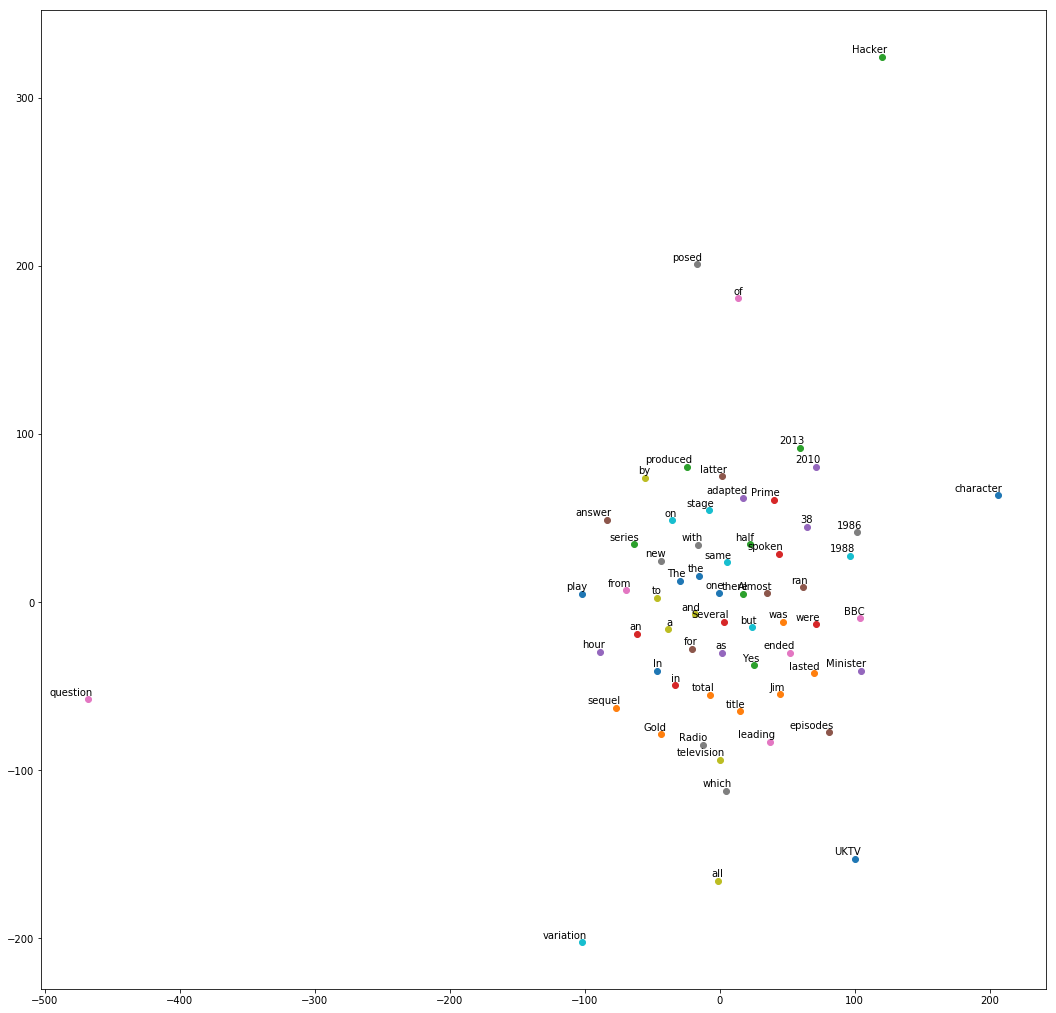• 年份
• 同一单词的大小写形式
• a 和 an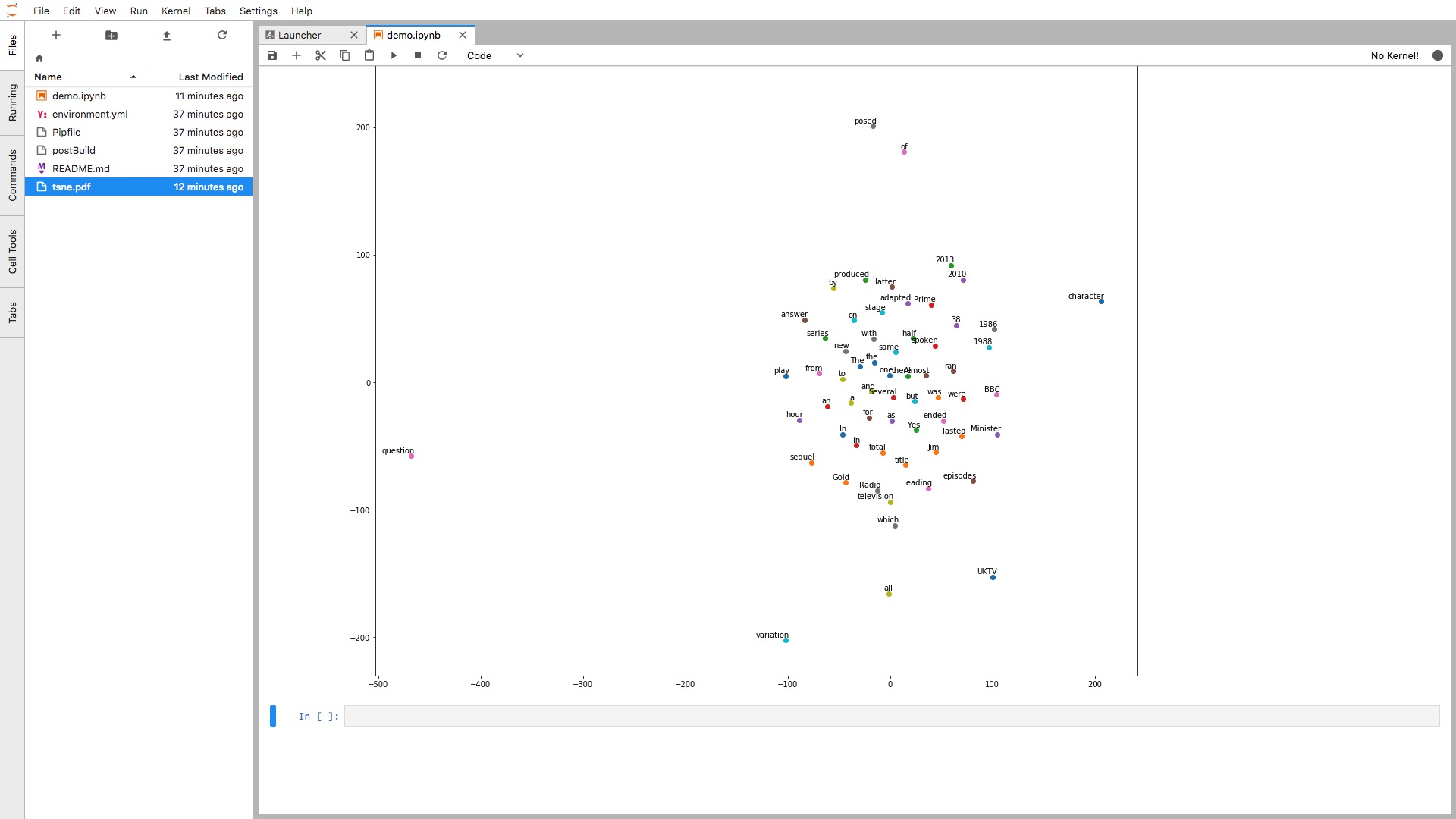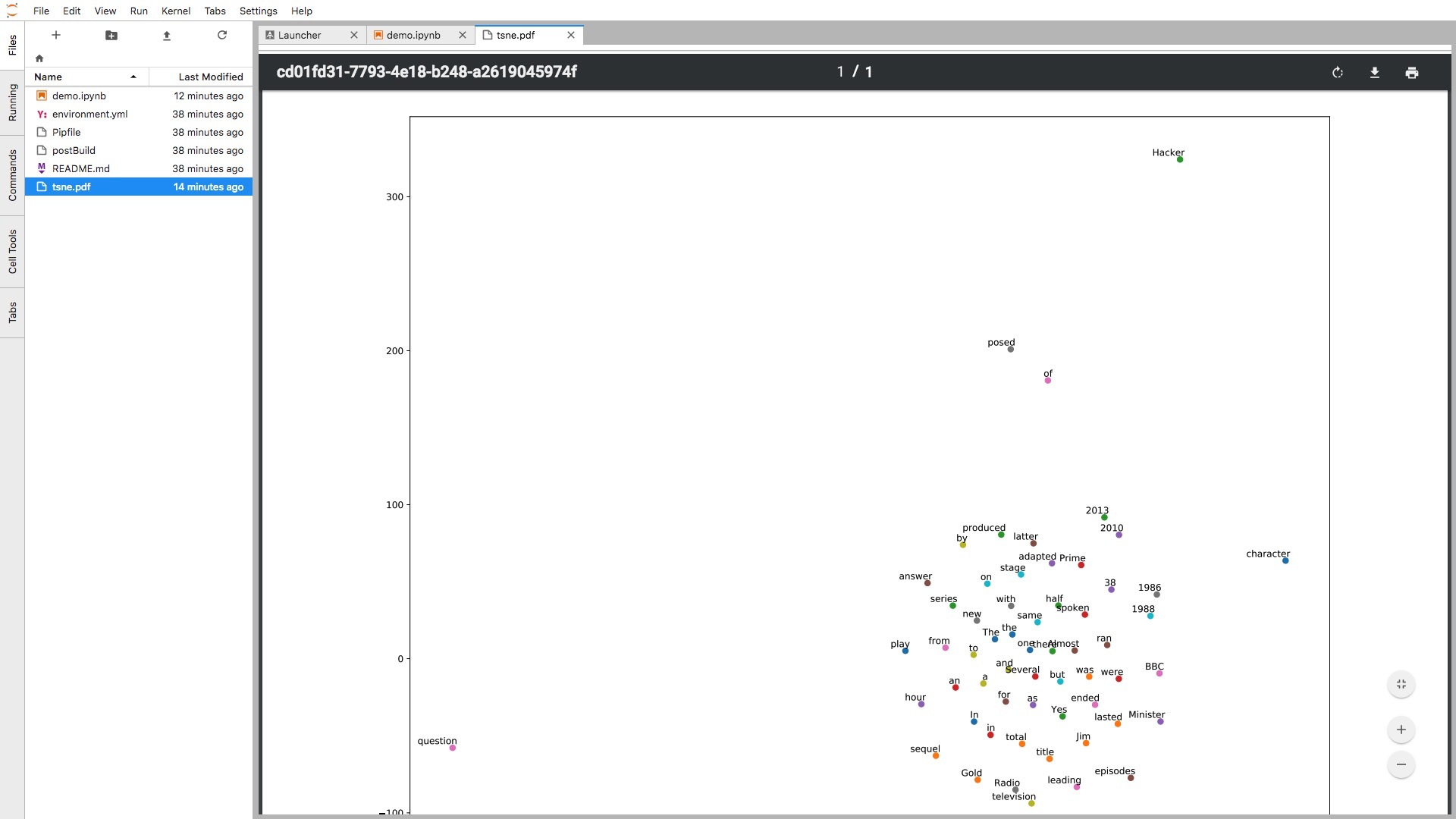# 源码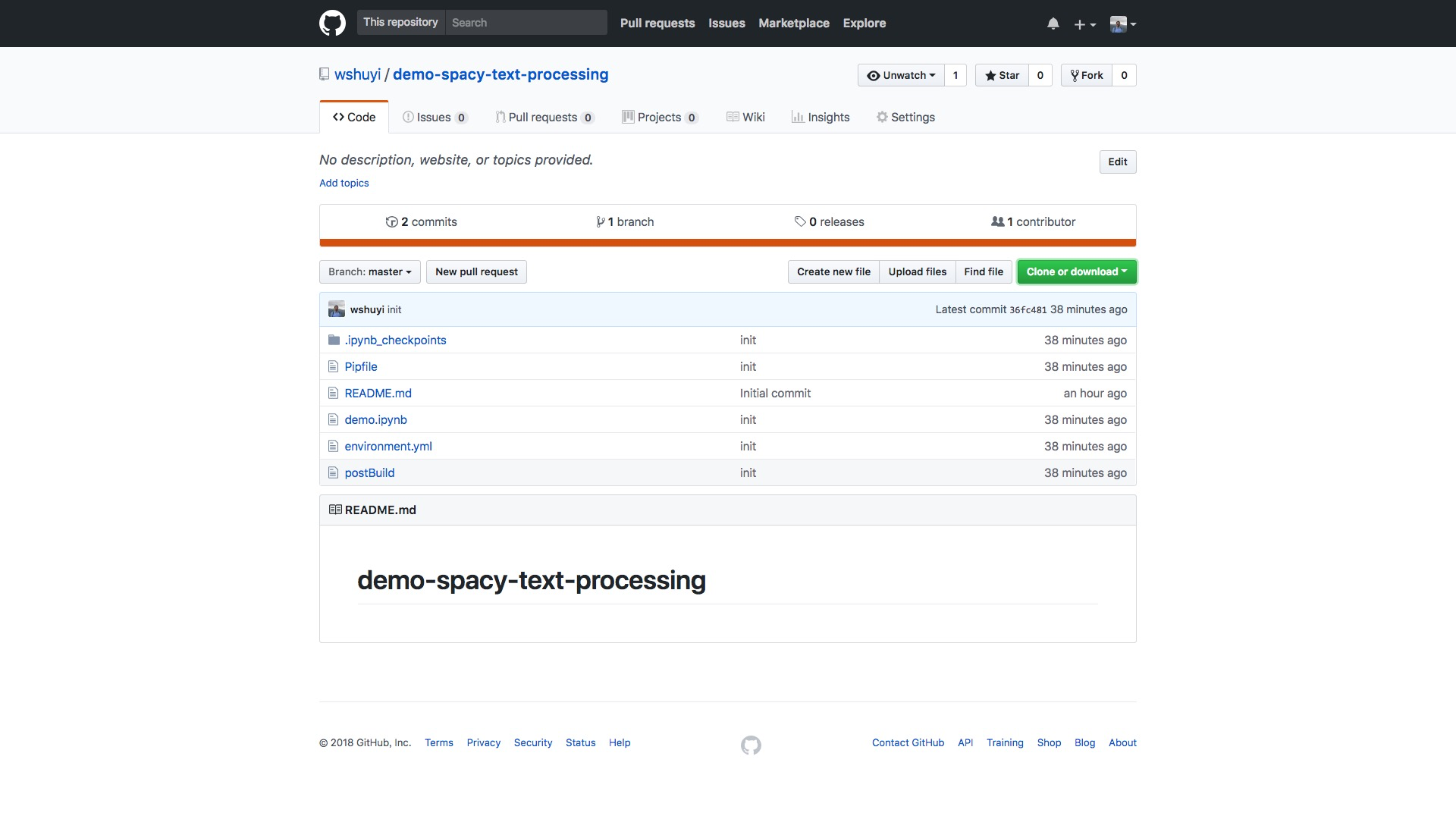• 词性分析
• 命名实体识别
• 依赖关系刻画
• 词嵌入向量的近似度计算
• 词语降维和可视化

+ 关注